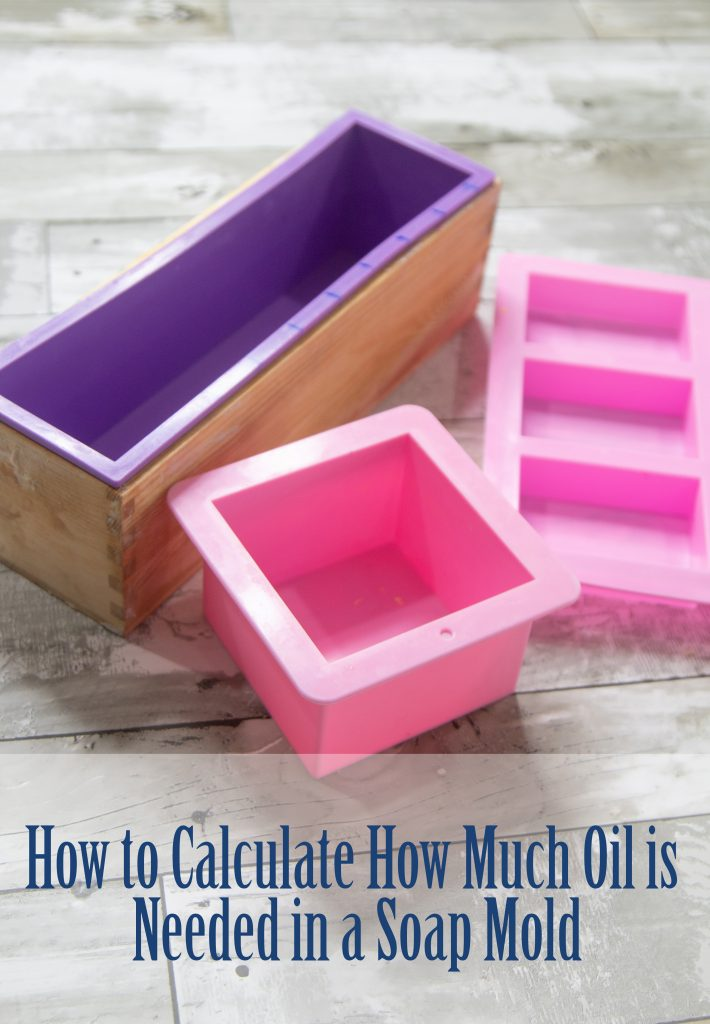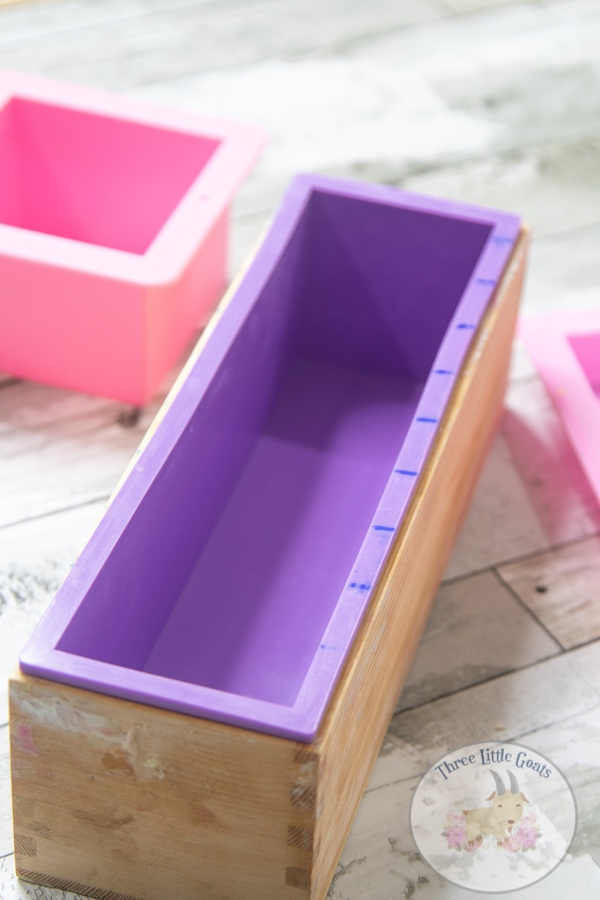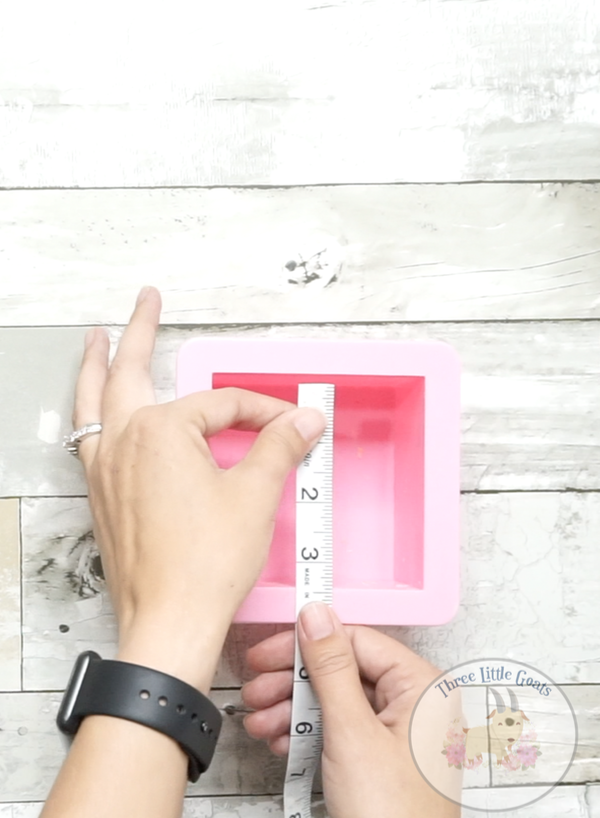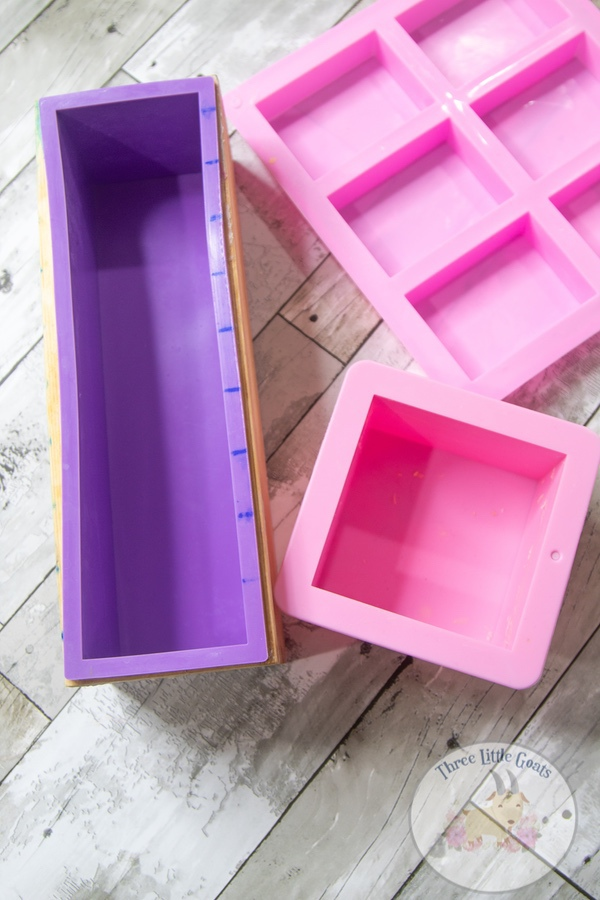Select Page

One of the biggest questions that I get is why I do not give out exact measurements of my soap recipes. And that is an easy question to answer: Because everyone uses different molds! So, today I am going to show you How to Calculate How Much Oil is Needed in a Soap Mold.

## How to Calculate How Much Oil is Needed in a Soap MoldIf I am using a 5lb soap mold, and you are using a 2lb soap mold, my measurements are going to be different than what you need. This is why you see a lot of soap recipes in percentages. If I use: 30% coconut oil, 30% Olive Oil, 30% Castor Oil, and 10% Shea Butter, you will be able to use those percentages to calculate the amounts you need for your mold. We will get into calculating soaping recipes next week, because first we need to know how much oil we will need for a particular soap mold.If you have a soap mold that states it holds 2 pounds of soap, it does not mean that you will need 2 pounds of oil. In fact, 2 pounds of oils will make way too much soap to fit into that mold. Instead we need to calculate exactly how much oil we will need for a particular mold.

### Calculating

First, we need to figure out the volume of the mold. We do this by measuring the INSIDE length, width, and depth of the mold. For example, the mold pictured below measures: 3.5 inches Long X 3.5 inches Wide X 2.5 inches Deep.When we multiply 3.5*3.5*2.5 we get: 30.6 oz. The 30.6 oz is the volume of the mold. This is how much soap can fit into the mold. In order to figure out how much oil we need, we need to take our math one step further, and multiply the volume by 40%. So, 30.6*.40 equals out to 12.24 oz. So, now I know that for this soap mold, I wll need 12.24 ounces of oil for my soap recipe.### Why 40%?

Some people will calculate by 39%, some will do 40%. As you become more experienced, that number may change even more. That will be discussed later when we talk more about water discounts and super fatting. The 40% consists of 35% for the water and 5% of the sodium hydroxide, because they need room in your mold too.  Below you will find a video explaining how to calculate how much oil is needed in a soap mold.

Be sure to subscribe to our YouTube Channel for more soaping videos!

Some of my go to soaping supplies: